## Friday, June 28, 2013

### Plots of x^t + y^t = n^t (x, y, n are integers)

Happy Friday almost July 2013!

This is a series of plots of the following:

1. x^2 + y^2 = n^2
2. x^2 + y^2 = n^3
3. x^3 + y^3 = n^2
4. x^3 + y^2 = n^2
5. x^3 + y^2 = n^3

For x, y, and n all integers. Plots are pointed for combinations where the result, n, is an integer. The range tested is 0 ≤ x ≤ 200 and 0 ≤ y ≤ 200. I used the TI nSpire CX CAS iOS app.

How many points?

1. x^2 + y^2 = n^2, 703 points
2. x^2 + y^2 = n^3, 55 points
3. x^3 + y^3 = n^2, 97 points
4. x^3 + y^2 = n^2, 375 points
5. x^3 + y^2 = n^3, 213 points, mostly when y=0

Program Outline: (for plot 1, similar format for the rest)
Define test()
Prgm
xs:={ }
ys:={ }
For k,0,200
For l,0,200
If iPart(√(k^2+l^2))=0 Then
xs:=augment(xs,{k})
ys:=augment(ys,{l})
EndIf
EndFor
EndFor
EndPrgm

And now to the fun part... Enjoy!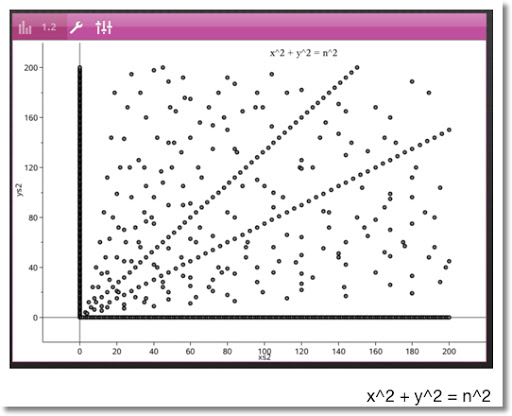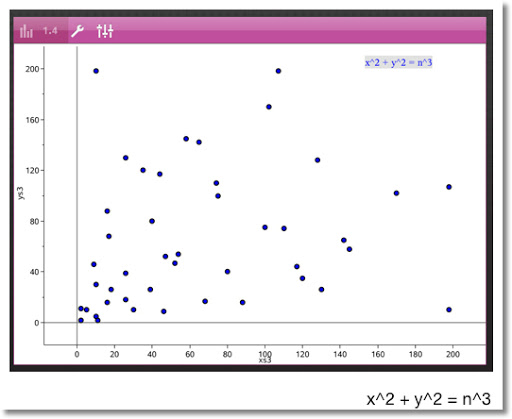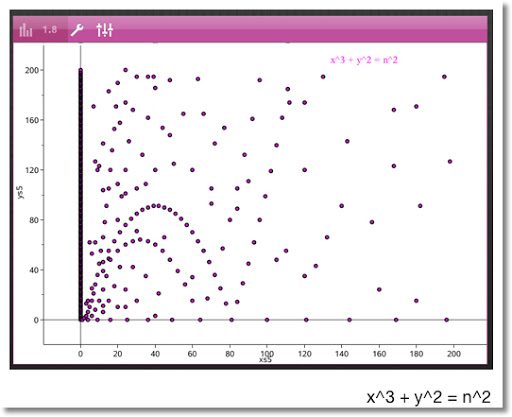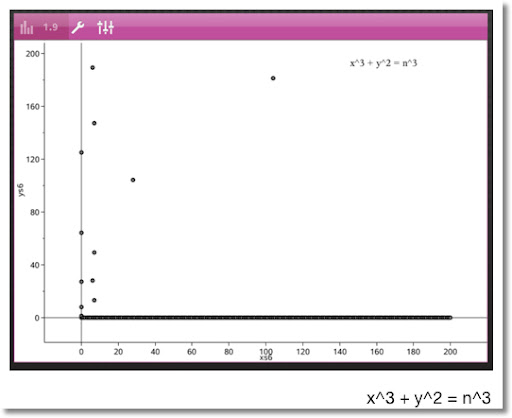That's it for now - as always thanks for your support and words of encouragement and your questions. :)

Eddie

This blog is property of Edward Shore. 2013

## Friday, June 21, 2013

### Integers: x^5 + y^5 = n^2, x^2 + y^2 = n^5, x^2 + y^3 = n^2 and x^3 + y^2 = n^3

Let x, y, and n be integers.

x^2 + y^2 = n^5

x and y tested: 1 ≤ x ≤ 30, 1 ≤ y ≤ 30

What I found: (there may be more outside the range I tested)
4^2 + 4^2 = 2^5
(Thank you Bill Zimmerly for pointing out my typo! (32 is not 5^3))

HP 32sii Program:

LBL E
x^2
x<>y
x^2
+
5
x√y
RTN

x^5 + y^5 = n^2

x and y tested: 1 ≤ x ≤ 30, 1 ≤ y ≤ 30

Again, I am looking for combinations where x, y, and n are all integers.

In the range tested I found:
2^5 + 2^5 = 8^2
8^5 + 8^5 = 256^2
18^5 + 18^5 = 1944^2

HP 32Sii Program:

LBL F
5
y^x
x<>y
5
y^x
+

RTN

The next program will cover two explorations. I will present the program first, then the equations.

HP32Sii Program:
LBL G
x^2
x<>y
3
y^x
+
ENTER
√ \\ √(x^2 + y^3)
R/S
x<>y
3
x√y \\cube root of (x^2 + y^3)
RTN

I used this program for two tests. The area tested was 1 ≤ x ≤ 16 and 1 ≤ y ≤ 16. I am looking for combinations where x, y, and n are integers.

x^2 + y^3 = n^2

2^3 + 1^2 = 3^2
3^3 + 3^2 = 6^2
4^3 + 6^2 = 10^2
5^3 + 10^2 = 15^2
6^3 + 3^2 = 15^2
8^3 + 8^2 = 24^2
12^3 + 6^2 = 42^2
12^3 + 11^2 = 43^2
3^3 + 13^2 = 14^2
4^3 + 15^2 = 17^2
6^3 + 15^2 = 21^2
10^3 + 15^2 = 35^2
15^3 + 15^2 = 60^2

x^2 + y^3 = n^3

7^3 + 13^2 = 60^2

Happy June Solstice!

Eddie

This blog is property of Edward Shore. 2013

## Sunday, June 16, 2013

### Integers: x^2 + y^2 = n^3, x^3 + y^3 = n^2, x^2 - y^2 = n^3, x^3 - y^3 = n^2

Let x, y, n be integers. I explore x and y for 1 through 15. I use an HP 32Sii to help me with the calculations.

x^2 + y^2 = n^3

2^2 + 2^2 = 2^3
2^2 + 11^2 = 5^3
5^2 + 10^2 = 5^3

Program:

LBL A
x^2
x<>y
x^2
+
3
1/x
y^x
RTN

x^3 + y^3 = n^2

1^3 + 2^3 = 3^2
2^3 + 2^3 = 4^2
8^3 + 4^3 = 24^2
8^3 + 8^3 = 32^2

Program:

LBL B
3
y^x
x<>y
3
y^x
+

RTN

x^2 - y^2 = n^3, x > y

15^2 - 10^2 = 5^3
15^2 - 3^2 = 6^3
14^2 - 13^2 = 3^3
10^2 - 6^2 = 4^3
6^2 - 3^2 = 3^3
3^2 - 1^2 = 2^3

Program:

LBL C
x^2
x<>y
x^2
x<>y
-
3
x√y
RTN

x^3 - y^3 = n^2, x > y

14^3 - 7^3 = 49^2
10^3 - 6^3 = 28^2
8^3 - 7^3 = 13^2

Program:

LBL D
3
y^x
x<>y
3
y^x
x<>y
-

RTN

If you want to find more integer triplets, happy exploring!

To all the dads out there - Happy Father's Day! To my dad, I am so proud of you!

Until next time,

Eddie

Happy One Week from the Summer Solstice!

This blog is property of Edward Shore. 2013

## Friday, June 7, 2013

### HP35S Music Pitch: Next Half-Step Up and Down

HP35S Music Pitch: Next Half-Step Up and Down

Source: "Sourcebook for Programmable Calculators" Texas Instruments (TI-58/59) - 1978 (,I believe, apologize if get the year wrong)

next pitch = original pitch * (twelfth root of 2) ≈ original pitch * 1.05946309436

Pitch is in HZ (Hertz)

256 Hz one octave lower than Middle C
512 Hz Middle C
1024 Hz one octave higher than Middle C

Steps: C C# D D# E F F# G G# A A# B

HP35S Program:
Next half-step up: pitch, XEQ M001
Next half-step down: pitch, XEQ M009
Repeat each step with R/S, stop whenever you like

M001 LBL M
M002 2
M003 12
M004 1/x
M005 y^x
M006 *
M007 R/S
M008 GTO M001
M009 2
M010 12
M011 1/x
M012 y^x
M013 ÷
M014 R/S
M015 GTO M009

Have fun and thanks for your support! Always appreciate the comments and followers! Eddie

This blog is property of Edward Shore. 2013

### HP 35S: Sun Altitude, Azimuth, Solar Pond Absorption

HP 35S: Sun Altitude, Azimuth, Solar Pond Absorption

Source: Sun Altitude, Azimuth, Solar Pond Absorption, HP 67/97 Energy Conservation December 1978, Author: HP

Input:

D = days after March 21 (later will be sun's declination : 23.45 sin (D * .9856°) )
L = latitude given in D.MMSS (degrees minutes seconds) format (avoid ±90°)
T = time before solar noon (12:00 PM), if the time is after noon, enter hours as a negative (example: 3:00 PM → -3)
N = index of refraction of surface/fluid (see below)

Index of Refraction for Common Objects:

Water: 1.33
Ice: 1.309
Glass: 1.52
Diamond: 2.42

Formulas: (Degrees Mode)

Sun Declination
D = 23.45 * sin( days after March 21 * .9856°)

Altitude of the Sun (H):
H = asin (cos L * cos D * cos (15 * T) + sin L * sin D)

Azimuth of the Sun (A): (degree from latitude ground wise north)
A = acos ( (sin H * sin L - sin D) ÷ (cos L * cos H) )

Fraction of the surface penetrated by the sun that hour (T):
T = 2 * n * (x^2 + y^2) * sin H * cos R
Where
R = asin (cos H ÷ n)
x = (cos R + n * sin H)^-1
y = (sin H + n * cos R)^-1

Example 1:

Input:
D = 68 (May 28), L = 46°, T = 4 (8:00 AM), N = 1.33 (water)

Output:
H (altitude) = 35.98991°
A (azimuth) = 84.40835°
F (fraction of coverage) = 0.95943

Example 2:

Input:
D = 90 (June 19), L = 23°, T = -3 (3:00 PM), N = 2.42 (diamond)

Output:
H = 48.81756°
A = 99.85903°
F = 0.82156

Program:

U001 LBL U
U002 INPUT D \\ declination
U003 DEG
U004 0.9856
U005 *
U006 SIN
U007 23.45
U008 *
U009 STO D \\ altitude
U010 COS
U011 INPUT L
U012 HMS→ \\ or → H
U013 STO L
U014 COS
U015 *
U016 INPUT T
U017 15
U018 *
U019 COS
U020 *
U021 RCL L
U022 SIN
U023 RCL D
U024 SIN
U025 *
U026 +
U027 ASIN
U028 STO H
U029 VIEW H \\ azimuth
U030 SIN
U031 RCL L
U032 SIN
U033 *
U034 RCL D
U035 SIN
U036 -
U037 RCL L
U038 COS
U039 RCL H
U040 COS
U041 *
U042 ÷
U043 ACOS
U044 STO A
U045 VIEW A

U046 RCL H \\ fraction
U047 COS
U048 INPUT N
U049 ÷
U050 ASIN
U051 STO R
U052 COS
U053 RCL H
U054 SIN
U055 RCL* N
U056 +
U057 1/x
U058 x^2
U059 RCL H
U060 SIN
U061 RCL R
U062 COS
U063 RCL* N
U064 +
U065 1/x
U066 x^2
U067 +
U068 RCL* N
U069 2
U070 *
U071 RCL H
U072 SIN
U073 *
U074 RCL R
U075 COS
U076 *
U077 STO F
U078 VIEW F
U079 RTN

This blog is property of Edward Shore. 2013

### HP35S Fraunhofer Diffraction - Spherical

HP35S Fraunhofer Diffraction - Spherical
Source: HP 67/97 Optics Pac, June 1978

This version takes advantage of the HP's integral function. The trade off is that two labels are required. On the plus side, this program can be typed directly into a 32Sii or 33S.

Labels: F (Main), G (Integral)

Variables:

D = diameter in microns (10^-6 meters)
L = wavelength of light in microns (10^-6 meters)
A = θ, angle of the slit, entered in degrees

Formulas:

X = π D ÷ L
W = X sin A
J = int(cos(T - W sin T) dT, 0, π)/π
I = (X^2 J ÷ W)^2

Output:

Bessel function of the first kind (J) - paused for 2 seconds;
Fraunhofer Intensity (I) - dimensionless - as I understand it, this is how intense the diffraction is

Programs:

LBL F
INPUT D
INPUT L
÷
π
*
STO X
INPUT A
STO A
SIN
*
STO W
0
π
FN= G
∫ FN dT
STO J \\ Bessel store in J for future use, if desired
PSE
PSE
RCL ÷ W
RCL X
x^2
*
x^2
RTN

LBL G
COS(T-W*SIN(T))/π \\ enter as an equation (press [EQN])
RTN

This blog is property of Edward Shore. 2013

## Sunday, June 2, 2013

### HP 35S: Spherical Triangle

(First draft?)

Formulas:
sin A / sin a = sin B / sin b = sin C / sin c
cos A = - cos B cos C + sin B sin C cos a
cos a = cos b cos c + sin b sin c cos A

A, B, C are angles formed by the great circles (the "lines" of the Spherical triangle). Note that A + B + C > 180°. a, b, and c measure the arc length of great circles as angles measured from the center of the sphere.

Source: http://www.krysstal.com/sphertrig.html

Program: Label S
Calculator: HP 35S

I am not sure if I covered all possible scenarios.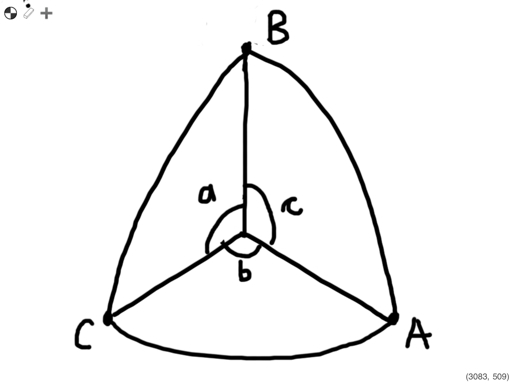Memory registers B and C are used for temporary purposes.

Given: B, A, b; Goal: a; Label S001
S001 LBL S
S002 SIN
S003 x<>y
S004 SIN
S005 ÷
S006 x<>y
S007 SIN
S008 ×
S009 ASIN
S010 RTN

Given: b, a, B; Goal: A; Label S011
S011 SIN
S012 x<>y
S013 SIN
S014 ×
S015 x<>y
S016 SIN
S017 ÷
S018 ASIN
S019 RTN

Given: a, b, c; Goal: A; Label S020
S020 STO C
S021 COS
S022 x<>y
S023 STO B
S024 COS
S025 ×
S026 x<>y
S027 COS
S028 x<>y
S029 -
S030 RCL B
S031 SIN
S032 RCL C
S033 SIN
S034 ×
S035 ÷
S036 ACOS
S037 RTN

Given: b, A, c; Goal: a; Label S038
S038 STO C
S039 SIN
S040 x<>y
S041 COS
S042 ×
S043 x<>y
S044 STO B
S045 SIN
S046 ×
S047 RCL C
S048 COS
S049 RCL B
S050 COS
S051 ×
S052 +
S053 ACOS
S054 RTN

Given: A, B, C; Find: a; Label S055
S055 STO C
S056 COS
S057 x<>y
S058 STO B
S059 COS
S060 ×
S061 x<>y
S062 COS
S063 +
S064 RCL B
S065 SIN
S066 RCL C
S067 SIN
S068 ×
S069 ÷
S070 ACOS
S071 RTN

Examples:

Given: B = 3.2145°, A = 2.2718°, b = 40°; XEQ S001; Result: a ≈ 65.4058°

Given: b = 60°, a = 40°, B = 4.95°; XEQ S011; Result A ≈ 3.6720°

Given: a = 4.11°, b = 5°, c = 6.03°; XEQ S020; Result A ≈ 42.5439°

Given: b = 3.996°, A = 49°, c = 6.314°; XEQ S038; Result a ≈ 4.7636°

Given: A = 124°, B = 45°, C = 76°; XEQ S055; Result a ≈ 124.4509°

Enjoy - hope this helps and have a great day!

Eddie

This blog is property of Edward Shore. 2013

### HP 35S: Planar Triangles

This program is set to solve common problems in plane (regular) triangles.

The programs can be adapted to any side lengths and angles necessary.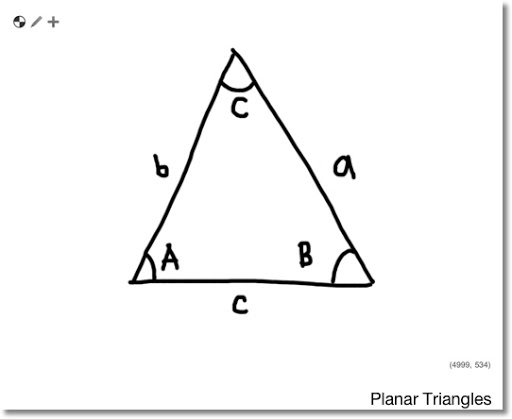Variables of Plane Triangles

Side length a with corresponding angle A,
Side length b with corresponding angle B, and
Side length c with corresponding angle C.

Labels and Stack Set Up: HP 35S

Angle-Angle-Side, Label P001, Stack: B, A, b, Goal: a

Side-Side-Angle, Label P009, Stack: b, a, B, Goal: A

Angle-Side-Angle, Label P015, Stack: b, A, c, Goal: a

Side-Side-Side, Label P033, Stack: a, b, c, Goal: A (angle corresponding to first side length entered)

* If you use a 15C, 32Sii, or other another RPN calculator, you will need to create four labels. The nice thing with the HP 35S is that you can create multiple programs within in one label. Memory registers B and C are temporary.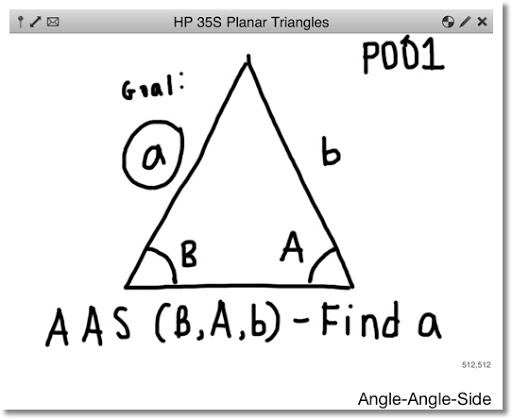Program P (Planar Triangles)

\\ Angle-Angle-Side: Law of Sines
\\ Stack: B, A, b; Find: a

P001 LBL P
P002 x<>y
P003 SIN
P004 ×
P005 x<>y
P006 SIN
P007 ÷
P008 RTN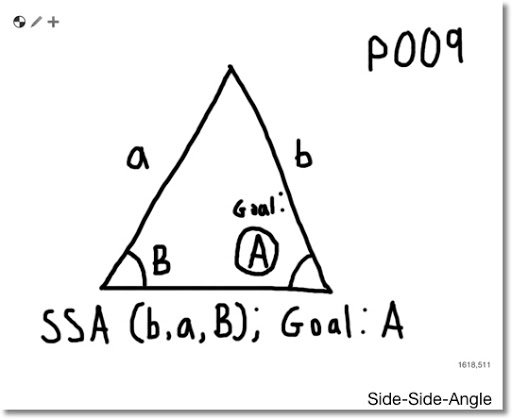\\ Side-Side-Angle: Law of Sines
\\ Stack: b, a, B; Find: A
P009 SIN
P010 ×
P011 x<>y
P012 ÷
P013 ASIN
P014 RTN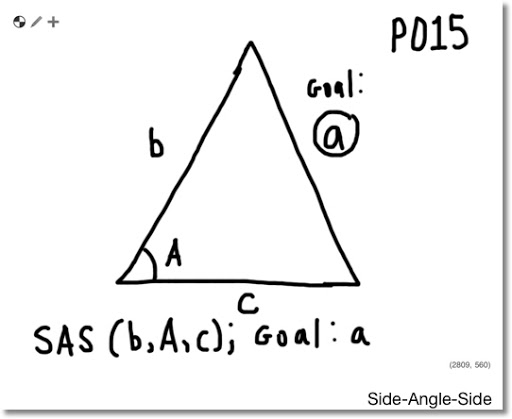\\ Side-Angle-Side: Law of Cosines
\\ Stack: b, A, c; Find: a
P015 STO C
P016 x<>y
P017 COS
P018 ×
P019 x<>y
P020 STO B
P021 ×
P022 2
P023 ×
P024 +/-
P025 RCL B
P026 x^2
P027 +
P028 RCL C
P029 x^2
P030 +
P031 √
P032 RTN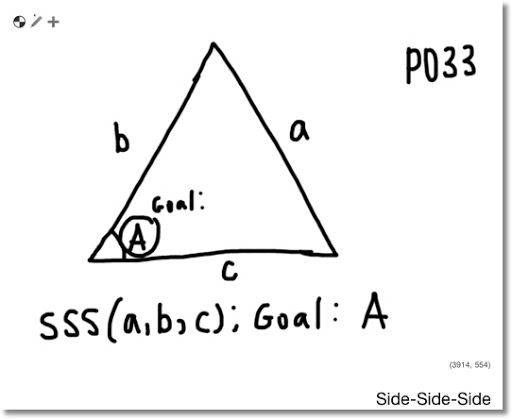\\ Side-Side-Side: Law of Cosines
\\ Stack: a, b, c; Find: A
P033 STO C
P034 x^2
P035 x<>y
P036 STO B
P037 x^2
P038 +
P039 x<>y
P040 x^2
P041 -
P042 2
P043 ÷
P044 RCL÷ B
P045 RCL÷ C
P046 ACOS
P047 RTN

Examples (Degrees Mode Used):
AAS: B = 30, A = 40, b = 4; a ≈ 5.1423

SSA: b = 5, a = 4, B = 90°; A ≈ 53.1301°

SAS: b = 8, A= 30°, c = 9; a ≈ 4.5047

SSS: a = 5, b = 4, c = 3; A = 90°

Hope this helps. I plan to post a program regarding spherical triangles.

Take care,

Eddie

This blog is property of Edward Shore. 2013

### TI-Nspire: Templates to Plot Functions, Parametric Equations, and Sequences

TI-Nspire:  Templates to Plot Functions, Parametric Equations, and Sequences Introduction The following are sample templates to plot functi...